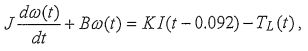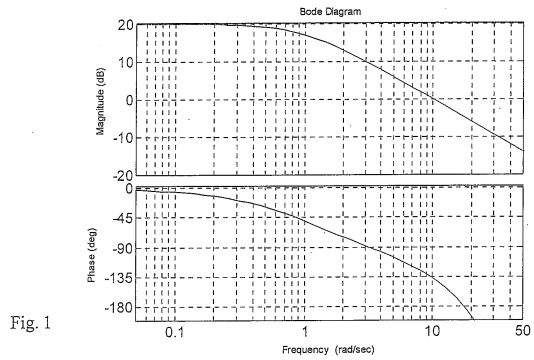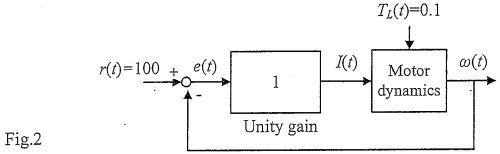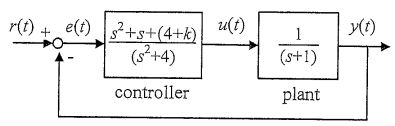【預購】摩檸客製化保溫瓶(粉)(藍)，歡迎下訂!
lvy>試卷(2022/08/05)

# 103 年 - 103 國立中山大學_碩士班招生考試_電機系(乙組)：控制系統#110068

【非選題】
1.

PROBLEM 1 (40%)
A DC motor drives a machine-tool spindle and workpiece, and its dynamic equation is described

bywhere ω is the angular velocity in units of rad/s, I is the input current, and Tt is the external torque generated by the cutting forces acting on the workpiece. There is an input delay of 0.092 s. The motor's torque constant K is found to be 0.1 by referring to the motor data sheet; but the moment of inertia J and the damping ratio B are unknown. Temporarily removing the workpiece (i.e., Tz=0), and doing the frequency response test by sweeping the frequency of the input current I, we can obtain the Bode plot of the transfer function between input current I and angular velocity w, as displayed in Fig. 1.【題組】 a.(20%) Estimate the values of J and B.

【非選題】
2.【題組】b. (20%) Suppose that we employ a unity feedback to control the motor anguiar velocity and set the desired angular velocity to 100 rad/s, as shown in Fig. 2. Assume the load torque is constant TL=0.1. Determine the phase margin of this feedback system. Also estimate the regulation error e(t) in steady state. y do(t)

【非選題】
3.

PROBLEM 2(15%)
Consider a system with the following unit step response y. Determine the poles and zeros of the system.【非選題】
4.

PROBLEM 3 (15%) Assume the operational amplifier in Fig. 3 is ideal. Show that the transfer function for the circuit can be written in the formDetermine the values of KP, KI, KD【非選題】
5.